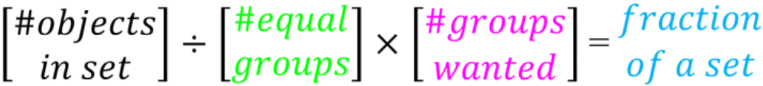# Fractions of a set#### Everything You Need in One Place

Homework problems? Exam preparation? Trying to grasp a concept or just brushing up the basics? Our extensive help & practice library have got you covered.#### Learn and Practice With Ease

Our proven video lessons ease you through problems quickly, and you get tonnes of friendly practice on questions that trip students up on tests and finals.#### Instant and Unlimited Help

Our personalized learning platform enables you to instantly find the exact walkthrough to your specific type of question. Activate unlimited help now!

0/1
##### Intros
###### Lessons
1. Introduction to Fractions of a Set:
How to find the fraction of a set of objects
0/11
##### Examples
###### Lessons
1. Picture models for fractions of a set
Picture models for fractions of a set
1. $\large \frac{1}{2}$ of 10 is ____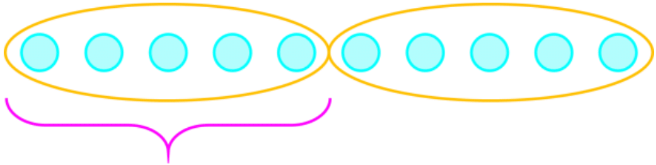2. ___ of 9 is 3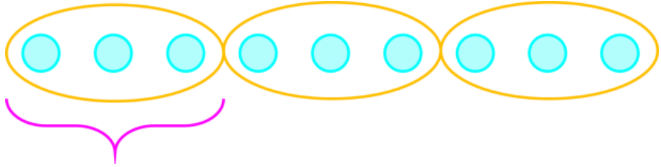2. Grouping circles for fractions of a set
For the set of circles, answer the sentence equation by:
• Drawing circles to make equal groups
• And then drawing a box around the given amount
1. $\large \frac{2}{3}$ of 12 is ____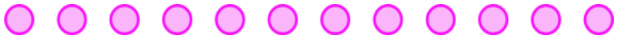2. $\large \frac{4}{5}$ of 15 is ____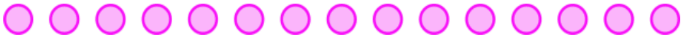3. Fractions of a set with division
Find fraction of the set and write as a division statement.
1. $\large \frac{1}{4}$ of 12 is ____
____ ÷ ____ = ____
2. $\large \frac{1}{2}$ of 18 is ____
____ ÷ ____ = ____
4. Fractions of a set with multiplication and division
Find fraction of the set and write a statement with both division and multiplication.
1. $\large \frac{2}{3}$ of 15 is ____
____ ÷ ____ × ____ = ____
2. $\large \frac{5}{6}$ of 24 is ____
____ ÷ ____ × ____ = ____
5. Word problems for fractions of a set
1. Raine has 20 socks. $\large \frac{3}{10}$ of the socks are yellow. How many socks are yellow?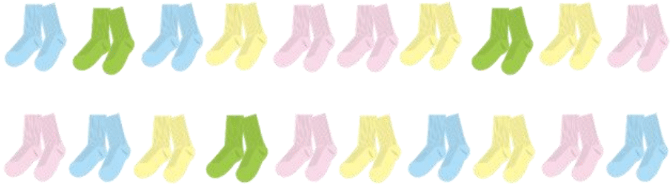2. 11 children are on the playground. $\large \frac{6}{11}$ of the children are boys. How many girls are on the playground?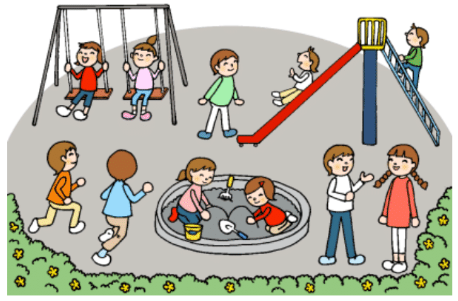3. Eli has 21 marbles. $\large \frac{2}{7}$ of the marbles are blue. How many not blue marbles does Eli have?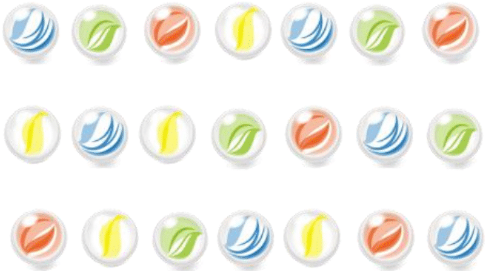0%
##### Practice
###### Free to Join!
StudyPug is a learning help platform covering math and science from grade 4 all the way to second year university. Our video tutorials, unlimited practice problems, and step-by-step explanations provide you or your child with all the help you need to master concepts. On top of that, it's fun - with achievements, customizable avatars, and awards to keep you motivated.
• #### Easily See Your ProgressWe track the progress you've made on a topic so you know what you've done. From the course view you can easily see what topics have what and the progress you've made on them. Fill the rings to completely master that section or mouse over the icon to see more details.
• #### Make Use of Our Learning Aids###### Practice Accuracy

Get quick access to the topic you're currently learning.

See how well your practice sessions are going over time.

Stay on track with our daily recommendations.

• #### Earn Achievements as You LearnMake the most of your time as you use StudyPug to help you achieve your goals. Earn fun little badges the more you watch, practice, and use our service.
• #### Create and Customize Your AvatarPlay with our fun little avatar builder to create and customize your own avatar on StudyPug. Choose your face, eye colour, hair colour and style, and background. Unlock more options the more you use StudyPug.
###### Topic Notes

In this lesson, we will learn:

• How to use fractions to talk about sets of objects
• How to use division and multiplication to solve for fraction of a set

Notes:

• We can use fractions to talk about sets of objects. We can use images or just simplify to a simple shape like a circle.
• Ex. If Austin has 8 jellybeans and he wants to give $\large \frac{1}{4}$ to her, how many jellybeans will he give?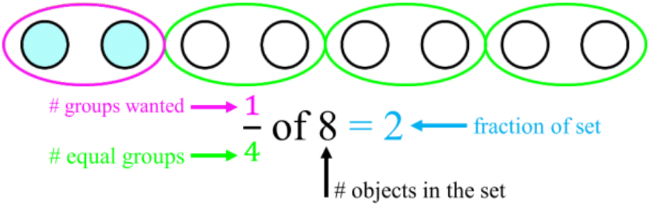• By making 4 equal groups and then giving just 1 of those groups, we realize that there is 2 in each group, so he gave his sister 2 jellybeans.
• We can use division and multiplication to find the fraction of a set
• Ex. After giving his sister jellybeans, Austin has $\large \frac{3}{4}$ of his 8 jellybeans left.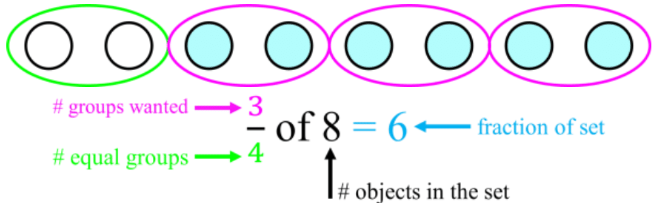• Step 1: divide 8 into 4 equal groups $\, \longrightarrow \,$ 8 ÷ 4 = 2
• Step 2: take the result and multiply by 3 groups $\, \longrightarrow \,$ 2 × 3 = 6
• The formula for a fraction of a set:
• following the format: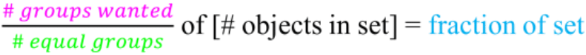• divide the denominator and multiply the numerator